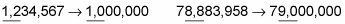##### Trigonometry Workbook For DummiesRounding numbers makes long numbers easier to work with. Here you will learn how to round numbers to the nearest ten, hundred, thousand, and beyond.

## Round numbers to the nearest ten

The simplest kind of rounding you can do is with two-digit numbers. When you round a two-digit number to the nearest ten, you simply bring it up or down to the nearest number that ends in 0. For example,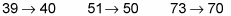Even though numbers ending in 5 are in the middle, always round them up to the next-highest number that ends in 0: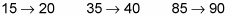Numbers in upper 90s get rounded up to 100: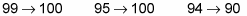After you know how to round a two-digit number, you can round just about any number. For example, to round most longer numbers to the nearest ten, just focus on the ones and tens digits: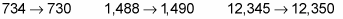Occasionally, a small change to the ones and tens digits affects the other digits. (This is a lot like when the odometer in your car rolls a bunch of 9s over to 0s.) For example: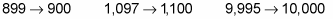## Round numbers to the nearest hundred and beyond

To round numbers to the nearest hundred, or thousand, or beyond, focus only on two digits: the digit in the place you’re rounding to and the digit to its immediate right. Change all other digits to the right of these two digits to 0s. For example, suppose you want to round 642 to the nearest hundred. Focus on the hundreds digit (6) and the digit to its immediate right (4):

642

These two digits are underlined. Now, just round these two digits as if you were rounding to the nearest ten, and change the digit to the right of them to a 0: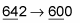Here are a few more examples of rounding numbers to the nearest hundred: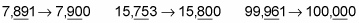When rounding numbers to the nearest thousand, underline the thousands digit and the digit to its immediate right. Round the number by focusing only on the two underlined digits and, when you’re done, change all digits to the right of these to 0s: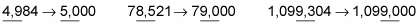Even when rounding to the nearest million, the same rules apply: